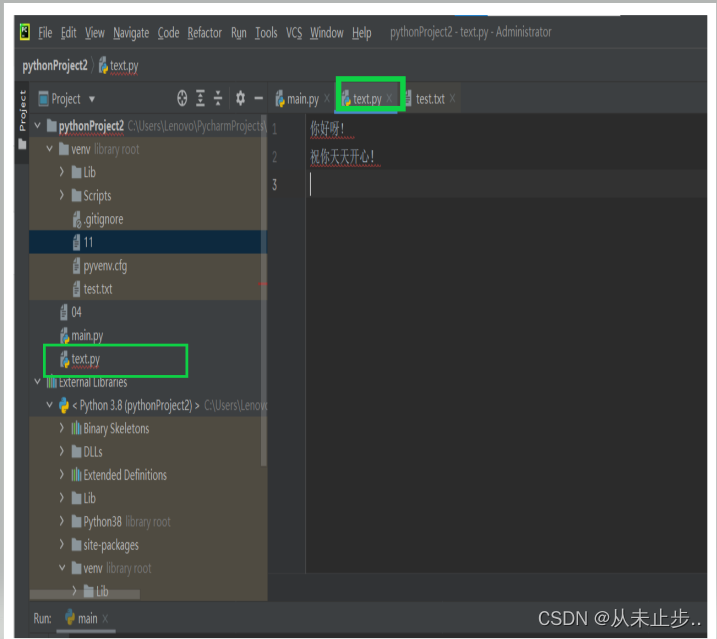﻿ Python常见文件操作的示例详解_python_脚本之家
python# Python常见文件操作的示例详解

## 从文件中读取数据

1：读取整个文件with open('text.py') as pythonProject2:#open(要打开的文件名称)
#open函数返回一个表示文件test.py的对象，python将该对象赋给pythonProject2
print(cc)python在当前执行的文件所在目录中查找指定的文件

## 为什么要提供文件路径with open(r"新建文本文档.txt",'r',encoding='utf-8') as f:
print(ff)with open(r"C:\Users\Lenovo\Desktop\新建文本文档.txt",'r',encoding='utf-8') as f:
print(ff)C:\path\to\file.txt


C:\\path\\to\\file.txt


## 逐行读取

for line in open("123.txt",encoding='utf-8'):
print(line)


for line in open(r"C:\Users\Lenovo\Desktop\新建文本文档.txt",encoding='utf-8'):
print(line)


f=open("123.txt",encoding='utf-8')
while line:
print(line)
f.close()


f=open(r"C:\Users\Lenovo\Desktop\新建文本文档.txt",encoding='utf-8')
while line:
print(line)
f.close()


with open("123.txt",encoding='utf-8') as f:
for line  in f:
print(line)


with open(r"C:\Users\Lenovo\Desktop\新建文本文档.txt",encoding='utf-8') as f:
for line in f:
print(line)


hi,python

print(line.rstrip())


hi,python

## 创建一个包含文件各行内容的列表

with open("123.txt",encoding='utf-8') as f:
//这样处理后，在with代码块外仍然可以使用变量lines
for line  in lines:
print(line)


hi,python

## 使用文件中的内容

with open("123.txt",encoding='utf-8') as f:
pi=str()#创建空的字符串用于存储文本内容
for line  in lines:
pi+=line.rstrip()#将每行末尾的空行去掉
print(pi)
print(len(pi))#输出字符串的长度


1234562345671038417968539
25with open("123.txt",encoding='utf-8') as f:
pi=int()#进行强制转化
#pi=float()
for line  in lines:
pi+=int(line)
#pi+=float(line)
print(pi)


1038418326562

## 包含千位以上的大型文件

with open("123.txt",encoding='utf-8') as f:
pi=str()#创建空的字符串用于存储文本内容
for line  in lines:
pi+=line.rstrip()#将每行末尾的空行去掉
print(f"{pi[:52]}.....")#为了避免显示的内容不停的滚动，只打印前52位
print(len(pi))#输出字符串的长度


1234562345671038417968539168519635885951538287826636.....
1596

## 圆周率中包含你的生日吗

with open("123.txt",encoding='utf-8') as f:
pi=str()#创建空的字符串用于存储文本内容
for line  in lines:
pi+=line.rstrip()#将每行末尾的空行去掉
you_birthday=str(input("请输入你的生日"))
if you_birthday in pi:
print("yes")
else:
print("NO")


yes

## 写入文件

1：写入空文件：

with open("123.txt",'w',encoding='utf-8') as f:
f.write("晚上好")#open(名称，操作模式 )#操作模式包括：w:写，r:读取 ，a:附加，r+:读写


python只能将字符串写入文本文件文件，要是想将数据存储到文本文件中，需要先用str（）将其转化为字符串格式。

with open("123.txt",'w',encoding='utf-8') as f:
f.write("早上好---good morning")
f.write("中午好---good afternoon")
f.write("晚上好---good evening")with open("123.txt",'w',encoding='utf-8') as f:
f.write("早上好---good morning\n")
f.write("中午好---good afternoon\n")
f.write("晚上好---good evening\n")## 附加到文件

with open("123.txt",'a',encoding='utf-8') as f:#以附加模式打开
f.write("hello,world")#将hello，world写入文件
`